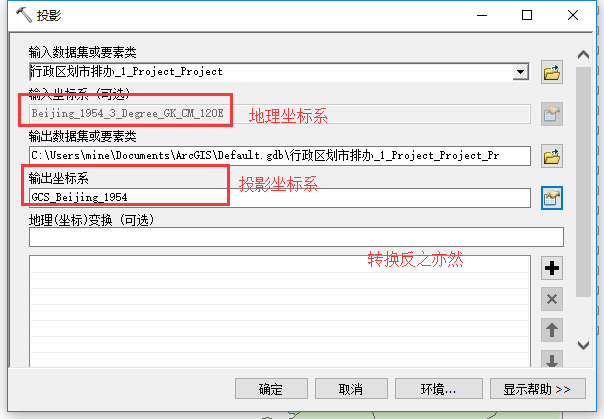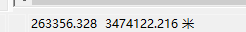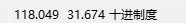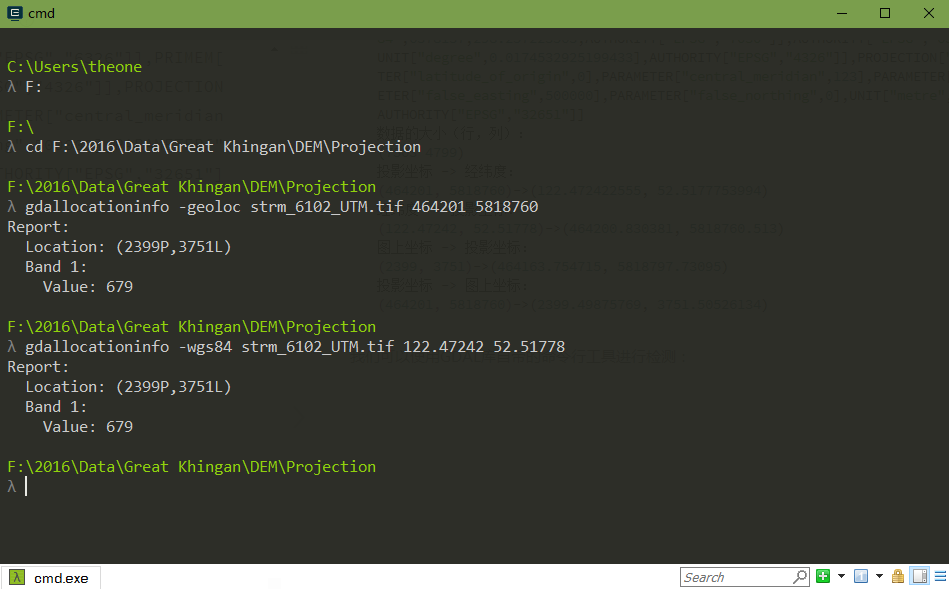• GDAL python读取shp进行影像投影坐标和地理坐标的转换 之前只在网上找到了使用gdal进行tif格式影像的投影坐标与地理坐标转换的代码，但没有专门针对shp格式的数据的教程。正巧现在需要用mongodb做矢量入库，建立...
之前只在网上找到了使用gdal进行tif格式影像的投影坐标与地理坐标转换的代码，但没有专门针对shp格式的数据的教程。正巧现在需要用mongodb做矢量入库，建立空间索引（2dshere）时必须用经纬度而不是投影坐标，就参考别人的代码做了一些修改，实现了shp的投影坐标转地理坐标。

参考博客地址：https://blog.csdn.net/theonegis/article/details/54427906

from osgeo import gdal
from osgeo import osr
from osgeo import ogr

#输入为待操作shp的路径，和一组待转换的投影坐标
def prj2geo(path,x,y):
# 为了支持中文路径，请添加下面这句代码
gdal.SetConfigOption("GDAL_FILENAME_IS_UTF8", "NO")
# 为了使属性表字段支持中文，请添加下面这句
gdal.SetConfigOption("SHAPE_ENCODING", "")
# 注册所有的驱动
ogr.RegisterAll()
# 数据格式的驱动
driver = ogr.GetDriverByName('ESRI Shapefile')
ds = driver.Open(path);
layer0 = ds_simple.GetLayerByIndex(0);

#或取到shp的投影坐标系信息
prosrs = layer0.GetSpatialRef()
geosrs = osr.SpatialReference()
#设置输出坐标系为WGS84
geosrs.SetWellKnownGeogCS("WGS84")

ct = osr.CoordinateTransformation(prosrs, geosrs)
coords = ct.TransformPoint(x, y)
#输出为转换好的经纬度
return coords[:2]


展开全文• 不同坐标系空间参考转换是一个繁杂的过程，常见的转换方式有七参数转换等，但是在相同坐标系下的地理坐标和投影坐标系可以直接进行转换转换过程 &nbsp;&nbsp;&nbsp;&nbsp;&gt;&gt;...
前言
不同坐标系空间参考转换是一个繁杂的过程，常见的转换方式有七参数转换等，但是在相同坐标系下的地理坐标系和投影坐标系可以直接进行转换。
转换过程
>>ArcToolBox / 数据管理工具 / 投影和变换 / 要素 / 投影这样就完成了投影坐标系和地理坐标系的转换注意:简单做法的前提是投影坐标系和地理坐标系处于相同的坐标系下。


展开全文ArcMap
• 对点坐标和数据集进行投影坐标和地理坐标系之间的转换
• 我使用GDAL库写了四个函数分别进行投影坐标与地理坐标（经纬度）之间的转换投影坐标和图上坐标（行列号）之间的转换。有需要的朋友可以参考。 直接上代码吧，因为代码很简单（Python版本）。# -*- encoding: utf-...
我使用GDAL库写了四个函数分别进行投影坐标与地理坐标（经纬度）之间的转换，投影坐标和图上坐标（行列号）之间的转换。有需要的朋友可以参考。
直接上代码吧，因为代码很简单（Python版本）。

# -*- encoding: utf-8 -*-

from osgeo import gdal
from osgeo import osr
import numpy as np

def getSRSPair(dataset):
'''
获得给定数据的投影参考系和地理参考系
:param dataset: GDAL地理数据
:return: 投影参考系和地理参考系
'''
prosrs = osr.SpatialReference()
prosrs.ImportFromWkt(dataset.GetProjection())
geosrs = prosrs.CloneGeogCS()
return prosrs, geosrs

def geo2lonlat(dataset, x, y):
'''
将投影坐标转为经纬度坐标（具体的投影坐标系由给定数据确定）
:param dataset: GDAL地理数据
:param x: 投影坐标x
:param y: 投影坐标y
:return: 投影坐标(x, y)对应的经纬度坐标(lon, lat)
'''
prosrs, geosrs = getSRSPair(dataset)
ct = osr.CoordinateTransformation(prosrs, geosrs)
coords = ct.TransformPoint(x, y)
return coords[:2]

def lonlat2geo(dataset, lon, lat):
'''
将经纬度坐标转为投影坐标（具体的投影坐标系由给定数据确定）
:param dataset: GDAL地理数据
:param lon: 地理坐标lon经度
:param lat: 地理坐标lat纬度
:return: 经纬度坐标(lon, lat)对应的投影坐标
'''
prosrs, geosrs = getSRSPair(dataset)
ct = osr.CoordinateTransformation(geosrs, prosrs)
coords = ct.TransformPoint(lon, lat)
return coords[:2]

def imagexy2geo(dataset, row, col):
'''
根据GDAL的六参数模型将影像图上坐标（行列号）转为投影坐标或地理坐标（根据具体数据的坐标系统转换）
:param dataset: GDAL地理数据
:param row: 像素的行号
:param col: 像素的列号
:return: 行列号(row, col)对应的投影坐标或地理坐标(x, y)
'''
trans = dataset.GetGeoTransform()
px = trans + col * trans + row * trans
py = trans + col * trans + row * trans
return px, py

def geo2imagexy(dataset, x, y):
'''
根据GDAL的六 参数模型将给定的投影或地理坐标转为影像图上坐标（行列号）
:param dataset: GDAL地理数据
:param x: 投影或地理坐标x
:param y: 投影或地理坐标y
:return: 影坐标或地理坐标(x, y)对应的影像图上行列号(row, col)
'''
trans = dataset.GetGeoTransform()
a = np.array([[trans, trans], [trans, trans]])
b = np.array([x - trans, y - trans])
return np.linalg.solve(a, b)  # 使用numpy的linalg.solve进行二元一次方程的求解

if __name__ == '__main__':
gdal.AllRegister()
dataset = gdal.Open(r"F:\2016\Data\Great Khingan\DEM\Projection\strm_6102_UTM.tif")
print('数据投影：')
print(dataset.GetProjection())
print('数据的大小（行，列）：')
print('(%s %s)' % (dataset.RasterYSize, dataset.RasterXSize))

x = 464201
y = 5818760
lon = 122.47242
lat = 52.51778
row = 2399
col = 3751

print('投影坐标 -> 经纬度：')
coords = geo2lonlat(dataset, x, y)
print('(%s, %s)->(%s, %s)' % (x, y, coords, coords))
print('经纬度 -> 投影坐标：')
coords = lonlat2geo(dataset, lon, lat)
print('(%s, %s)->(%s, %s)' % (lon, lat, coords, coords))

print('图上坐标 -> 投影坐标：')
coords = imagexy2geo(dataset, row, col)
print('(%s, %s)->(%s, %s)' % (row, col, coords, coords))
print('投影坐标 -> 图上坐标：')
coords = geo2imagexy(dataset, x, y)
print('(%s, %s)->(%s, %s)' % (x, y, coords, coords))


输出结果：

数据投影：
PROJCS["WGS_1984_UTM_Zone_51N",GEOGCS["WGS 84",DATUM["WGS_1984",SPHEROID["WGS 84",6378137,298.257223563,AUTHORITY["EPSG","7030"]],AUTHORITY["EPSG","6326"]],PRIMEM["Greenwich",0],UNIT["degree",0.0174532925199433],AUTHORITY["EPSG","4326"]],PROJECTION["Transverse_Mercator"],PARAMETER["latitude_of_origin",0],PARAMETER["central_meridian",123],PARAMETER["scale_factor",0.9996],PARAMETER["false_easting",500000],PARAMETER["false_northing",0],UNIT["metre",1,AUTHORITY["EPSG","9001"]],AUTHORITY["EPSG","32651"]]
数据的大小（行，列）：
(7503 4799)
投影坐标 -> 经纬度：
(464201, 5818760)->(122.472422555, 52.5177753994)
经纬度 -> 投影坐标：
(122.47242, 52.51778)->(464200.830381, 5818760.513)
图上坐标 -> 投影坐标：
(2399, 3751)->(464163.754715, 5818797.73095)
投影坐标 -> 图上坐标：
(464201, 5818760)->(2399.49875769, 3751.50526134)

注：关于投影坐标和图上坐标转换的六参数模型可以参考我的另外一篇博文：经纬度坐标和投影坐标的转换，其实质就是一个仿射变换。

我们可以使用GDAL库自带的命令行工具（gdallocationinfo）进行检测：其中参数-geoloc表示的后面给定坐标是投影坐标，-wgs84表示是WGS84参考系下的地理坐标（经纬度）。其输出是对应的图上坐标（行列号）。
具体参数可以使用gdallocationinfo –help查看。
展开全文GDAL Python
• 使用GDAL库写了四个函数分别进行投影坐标与地理坐标（经纬度）之间的转换投影坐标和图上坐标（行列号）之间的转换。有需要的朋友可以参考。 直接上代码吧，因为代码很简单（Python版本）。 # -*- encoding: utf-...
使用GDAL库写了四个函数分别进行投影坐标与地理坐标（经纬度）之间的转换，投影坐标和图上坐标（行列号）之间的转换。有需要的朋友可以参考。
直接上代码吧，因为代码很简单（Python版本）。

# -*- encoding: utf-8 -*-

from osgeo import gdal
from osgeo import osr
import numpy as np

def getSRSPair(dataset):
'''
获得给定数据的投影参考系和地理参考系
:param dataset: GDAL地理数据
:return: 投影参考系和地理参考系
'''
prosrs = osr.SpatialReference()
prosrs.ImportFromWkt(dataset.GetProjection())
geosrs = prosrs.CloneGeogCS()
return prosrs, geosrs

def geo2lonlat(dataset, x, y):
'''
将投影坐标转为经纬度坐标（具体的投影坐标系由给定数据确定）
:param dataset: GDAL地理数据
:param x: 投影坐标x
:param y: 投影坐标y
:return: 投影坐标(x, y)对应的经纬度坐标(lon, lat)
'''
prosrs, geosrs = getSRSPair(dataset)
ct = osr.CoordinateTransformation(prosrs, geosrs)
coords = ct.TransformPoint(x, y)
return coords[:2]

def lonlat2geo(dataset, lon, lat):
'''
将经纬度坐标转为投影坐标（具体的投影坐标系由给定数据确定）
:param dataset: GDAL地理数据
:param lon: 地理坐标lon经度
:param lat: 地理坐标lat纬度
:return: 经纬度坐标(lon, lat)对应的投影坐标
'''
prosrs, geosrs = getSRSPair(dataset)
ct = osr.CoordinateTransformation(geosrs, prosrs)
coords = ct.TransformPoint(lon, lat)
return coords[:2]

def imagexy2geo(dataset, row, col):
'''
根据GDAL的六参数模型将影像图上坐标（行列号）转为投影坐标或地理坐标（根据具体数据的坐标系统转换）
:param dataset: GDAL地理数据
:param row: 像素的行号
:param col: 像素的列号
:return: 行列号(row, col)对应的投影坐标或地理坐标(x, y)
'''
trans = dataset.GetGeoTransform()
px = trans + col * trans + row * trans
py = trans + col * trans + row * trans
return px, py

def geo2imagexy(dataset, x, y):
'''
根据GDAL的六 参数模型将给定的投影或地理坐标转为影像图上坐标（行列号）
:param dataset: GDAL地理数据
:param x: 投影或地理坐标x
:param y: 投影或地理坐标y
:return: 影坐标或地理坐标(x, y)对应的影像图上行列号(row, col)
'''
trans = dataset.GetGeoTransform()
a = np.array([[trans, trans], [trans, trans]])
b = np.array([x - trans, y - trans])
return np.linalg.solve(a, b)  # 使用numpy的linalg.solve进行二元一次方程的求解

if __name__ == '__main__':
gdal.AllRegister()
dataset = gdal.Open(r"F:\2016\Data\Great Khingan\DEM\Projection\strm_6102_UTM.tif")
print('数据投影：')
print(dataset.GetProjection())
print('数据的大小（行，列）：')
print('(%s %s)' % (dataset.RasterYSize, dataset.RasterXSize))

x = 464201
y = 5818760
lon = 122.47242
lat = 52.51778
row = 2399
col = 3751

print('投影坐标 -> 经纬度：')
coords = geo2lonlat(dataset, x, y)
print('(%s, %s)->(%s, %s)' % (x, y, coords, coords))
print('经纬度 -> 投影坐标：')
coords = lonlat2geo(dataset, lon, lat)
print('(%s, %s)->(%s, %s)' % (lon, lat, coords, coords))

print('图上坐标 -> 投影坐标：')
coords = imagexy2geo(dataset, row, col)
print('(%s, %s)->(%s, %s)' % (row, col, coords, coords))
print('投影坐标 -> 图上坐标：')
coords = geo2imagexy(dataset, x, y)
print('(%s, %s)->(%s, %s)' % (x, y, coords, coords))


输出结果：

数据投影：
PROJCS["WGS_1984_UTM_Zone_51N",GEOGCS["WGS 84",DATUM["WGS_1984",SPHEROID["WGS 84",6378137,298.257223563,AUTHORITY["EPSG","7030"]],AUTHORITY["EPSG","6326"]],PRIMEM["Greenwich",0],UNIT["degree",0.0174532925199433],AUTHORITY["EPSG","4326"]],PROJECTION["Transverse_Mercator"],PARAMETER["latitude_of_origin",0],PARAMETER["central_meridian",123],PARAMETER["scale_factor",0.9996],PARAMETER["false_easting",500000],PARAMETER["false_northing",0],UNIT["metre",1,AUTHORITY["EPSG","9001"]],AUTHORITY["EPSG","32651"]]
数据的大小（行，列）：
(7503 4799)
投影坐标 -> 经纬度：
(464201, 5818760)->(122.472422555, 52.5177753994)
经纬度 -> 投影坐标：
(122.47242, 52.51778)->(464200.830381, 5818760.513)
图上坐标 -> 投影坐标：
(2399, 3751)->(464163.754715, 5818797.73095)
投影坐标 -> 图上坐标：
(464201, 5818760)->(2399.49875769, 3751.50526134)

注：关于投影坐标和图上坐标转换的六参数模型可以参考我的另外一篇博文：经纬度坐标和投影坐标的转换，其实质就是一个仿射变换。

我们可以使用GDAL库自带的命令行工具（gdallocationinfo）进行检测：其中参数-geoloc表示的后面给定坐标是投影坐标，-wgs84表示是WGS84参考系下的地理坐标（经纬度）。其输出是对应的图上坐标（行列号）。
具体参数可以使用gdallocationinfo –help查看。

展开全文gdal python
• arcgis中将地理坐标转换投影坐标 / 经纬度坐标转换 1.导入需要转换的数据，以csv格式的点为例 (1) File-> Add Data -> Add XY Data… (2) 选择导入的数据后将经纬度坐标分别赋予“X Field”“Y Field”. 2...arcgis
• 我使用GDAL库写了四个函数分别进行投影坐标与地理坐标（经纬度）之间的转换投影坐标和图上坐标（行列号）之间的转换。有需要的朋友可以参考。 直接上代码吧，因为代码很简单（Python版本）。 # -*- encoding: utf-...
• dll中的方法及其参数有详细描述。 支持地理坐标投影坐标转换，以及投影坐标地理坐标转换。 主要输入参数为WKID。 如地理坐标系WGS1984的4326转成投影坐标系Beijing1954的2433
• 前言：原作者提供了js版本的坐标转换函数，和三种椭球体参数（北京54、西安80、WGS84），个人在百度上搜索了CGC2000坐标系的椭球参数，添加到函数里，使用java写的转换函数。在ArcMap里使用同一位置的两个不同坐标系...
• 通常需要将经纬度坐标与投影坐标相互转换，下面是一些源码，在ArcEngine中实现。 投影转经纬度 private IPoint PRJtoGCS(double x, double y) { IPoint pPoint = new PointClass(); pPoint.PutCoords(x...
• 0 相关名词 ...地理坐标系统（Geographic Coordinate System，GCS）1，坐标系是地心坐标系，用经纬度表示球面上的点。 世界大地测量系统（World Geodetic System, WGS），比如WGS84，是一种地理坐标系统。...GCS
• 现在好多人在使用ArcObject的时候都可能需要作经纬度平面坐标的相互转换。由于经纬度是球面坐标，平面坐标是X-Y的笛卡尔坐标系统，所以这是一个看起来比较难的问题。 #此前在首页部分显示#   好多人一上来就搬...
• 参考：http://blog.csdn.net/theonegis/article/details/54427906# -*- encoding: utf-8 -*-from osgeo import gdal from osgeo import osr import numpy as npdef getSRSPair... 获得给定数据的投影参考系和地理参考系gdal
• 坐标转换工具，能够进行地理坐标和各种投影之间的良好转换，可以一个一个转化，并支持批量转换。
• 所谓动态投影指，ArcMap中的Data 的空间参考或是说坐标系统是默认为第一加载到当前工作区的那个文件的坐标系统，后加入的数据，如果当前工作区坐标系统不相同，则ArcMap会自动做投影变换，把后加入的数据投影变换...
• 下面例子表示将地理坐标系下的影像DS1102-2102DF041_rec_geocoding_1s_match.tif转换投影坐标系下， 1 地理坐标系下的Map Info： 2 .1 找到Map下的Convert Map Projection工具，输入所需要转换坐标系的影像， ...envi
• 文章目录经纬度坐标系平面投影坐标地理坐标系 / 大地坐标系平面投影坐标系墨卡托投影法变量常量定义（1）墨卡托投影正解算公式（2）墨卡托投影逆解算公式Python代码实现（1）墨卡托投影正解算公式（2）墨卡托...
• 所谓动态投影，是指arcmap中的data的空间参考或是说坐标系统是默认为第一个加载到当前数据框的哪个文件的坐标系统，随后添加的数据，如果当前数据框的坐标系不一致，arcmap会自动做投影变化，把后添加的数据投影...
• 地理坐标转换投影坐标的过程可理解为投影。（投影：将不规则的地球曲面转换为平面） 2、地理坐标系 2.1 地球的三级逼近 2.1.1大地水准面 地球的自然表面有高山也有洼地，是崎岖不平的，我们要使用数学法则来...
• 简单介绍arcgis软件中坐标系定义、设置常见问题。
• 有很多三维GIS开发，需要使用屏幕坐标和地理坐标转换，如开发当前相机的状态栏： 这里，我们将介绍ArcGIS和SuperMap的屏幕坐标与地理坐标之间转换的调用接口。 SuperMap 使用Scene.PixelToGlobe可以实现屏幕坐标...
• 最近运用到QGis的开发，通过gdal库（操作各种栅格地理数据格式的库）解析shp文件得到想要的地理数据，开发过程中，想得到某段道路的折线长度，网上找很多资料都没有类似的代码示例（主要是讲解地理坐标和投影坐标的......